## Laplace Expansions for the Determinant

Using the definition of the determinant, the following expression was derived in Example 5: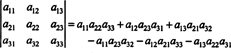This equation can be rewritten as follows:Each term on the right has the following form: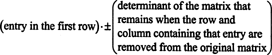In particular, note that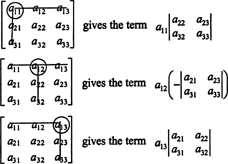If A = [ a ij ] is an n x n matrix, then the determinant of the ( n − 1) x ( n − 1) matrix that remains once the row and column containing the entry a ij are deleted is called the a ij minor, denoted mnr( a ij ). If the a ij minor is multiplied by (−1) i + j , he result is called the a ij cofactor, denoted cof( a ij ). That is,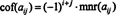Using this terminology, the equation given above for the determinant of the 3 x 3 matrix A is equal to the sum of the products of the entries in the first row and their cofactors: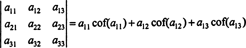This is called the Laplace expansion by the first row. It can also be shown that the determinant is equal to the Laplace expansion by the second row,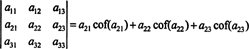or by the third row,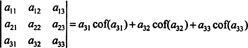Even more is true. The determinant is also equal to the Laplace expansion by the first columnby the second column, or by the third column. Although the Laplace expansion formula for the determinant has been explicitly verified only for a 3 x 3 matrix and only for the first row, it can be proved that the determinant of any n x n matrix is equal to the Laplace expansion by any row or any column.

Example 1: Evaluate the determinant of the following matrix using the Laplace expansion by the second column:The entries in the second column are a 12 = −1, a 22 = 2, and a 32 = 0. The minors of these entries, mnr( a 12), mnr( a 22), and mnr( a 32), are computed as follows:Since the cofactors of the second‐column entries are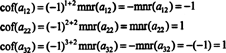the Laplace expansion by the second column becomesNote that it was unnecessary to compute the minor or the cofactor of the (3, 2) entry in A, since that entry was 0. In general, then, when computing a determinant by the Laplace expansion method, choose the row or column with the most zeros. The minors of those entries need not be evaluated, because they will contribute nothing to the determinant.

The factor (−1) i + j which multiplies the a ij minor to give the a ij cofactor leads to a checkerboard pattern of signs; each sign gives the value of this factor when computing the a ij cofactor from the a ij minor. For example, the checkerboard pattern for a 3 x 3 matrix looks like this:For a 4 x 4 matrix, the checkerboard has the formand so on.

Example 2: Compute the determinant of the following matrix: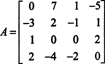First, find the row or column with the most zeros. Here, it's the third row, which contains two zeros; the Laplace expansion by this row will contain only two nonzero terms. The checkerboard pattern displayed above for a 4 by 4 matrix implies that the minor of the entry a 31 = 1 will be multiplied by +1, and the minor of the entry a 34 = 2 will be multiplied by −1 to give the respective cofactors: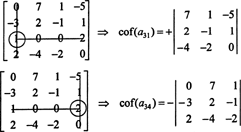Now, each of these cofactors—which are themselves determinants—can be evaluated by a Laplace expansion. Expanding by the third column,The other cofactor is evaluated by expanding along its first row: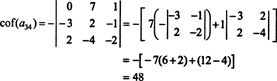Therefore, evaluating det A by the Laplace expansion along A's third row yieldsExample 3: The cross product of two 3‐vectors, x = x 1 i + x 2 j + x 3 k and y = y 1 i + y 2 j + y 3 k, is most easily evaluated by performing the Laplace expansion along the first row of the symbolic determinant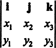This expansion givesTo illustrate, the cross product of the vectors x = 3 j − 3 k and y = −2 i + 2 jk is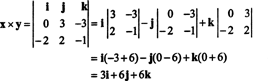Example 4: Is there a connection between the determinant of A T and the determinant of A?

In the 2 by 2 case, it is easy to see that det ( A T) = det A:In the 3 by 3 case, the Laplace expansion along the first row of A gives the same result as the Laplace expansion along the first column of A T, implying that det ( A T) = det A: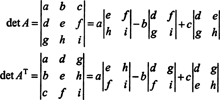Starting with the expansionfor the determinant, it is not difficult to give a general proof that det ( A T) = det A.

Example 5: Apply the result det ( A T) = det A to evaluategiven that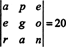(where a, e, g, n, o, p, and r are scalars).

Since one row exchange reverses the sign of the determinant (Property 2), two-row exchanges,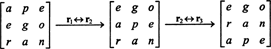will leave the determinant unchanged:But the determinant of a matrix is equal to the determinant of its transpose, soTherefore,Example 7: Given that the numbers 1547, 2329, 3893, and 4471 are all divisible by 17, prove that the determinant of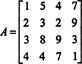is also divisible by 17 without actually evaluating it.

Because of the result det ( A T) = det A, every property of the determinant which involves the rows of A implies another property of the determinant involving the columns of A. For example, the determinant is linear in each column, reverses sign if two columns are interchanged, is unaffected if a multiple of one column is added to another column, and so on.

To begin, multiply the first column of A by 1000, the second column by 100, and the third column by 10. The determinant of the resulting matrix will be 1000·100·10 times greater than the determinant of A:Next, add the second, third, and fourth columns of this new matrix to its first column. None of these column operations changes the determinant; thus,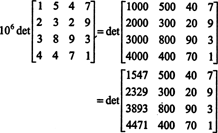Since each entry in the first column of this latest matrix is divisible by 17, every term in the Laplace expansion by the first column will be divisible by 17, and thus the sum of these terms—which gives the determinant—will be divisible by 17. Since 17 divides 10 6 det A, 17 must divide det A because 17 is prime and doesn't divide 10 6.

Example 7: A useful concept in higher‐dimensional calculus (in connection with the change‐of‐variables formula for multiple integrals, for example) is that of the Jacobian of a mapping. Let x and y be given as functions of the independent variables u and v:The Jacobian of the map ( u, v) ↦ ( x, y), a quantity denoted by the symbol δ( x, y)/δ( u, v), is defined to be the following determinant:To illustrate, consider the polar coordinate transformation,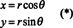The Jacobian of this mapping, ( r, θ) ↦ ( x, y), isThe fact that the Jacobian of this transformation is equal to r accounts for the factor of r in the familiar formula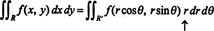where R′ is the region in the r−θ plane mapped by (*) to the region of integration R in the x−y plane.

The Jacobian can also be extended to three variables. For example, a point in 3‐space can be specified by giving its spherical coordinates—ϕ, and θ—which are related to the usual rectangular coordinates— x, y, and z—by the equations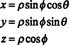See Figure .Figure 1

The Jacobian of the mapping (ρ, ϕ, θ) ↦ ( x, y, z) is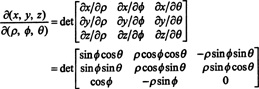By a Laplace expansion along the third row,The fact that the Jacobian of this transformation is equal to ρ 2 sin ϕ accounts for the factor of ρ 2 sin ϕ in the formula for changing the variables in a triple integral from rectangular to spherical coordinates:Laplace expansions following row‐reduction. The utility of the Laplace expansion method for evaluating a determinant is enhanced when it is preceded by elementary row operations. If such operations are performed on a matrix, the number of zeros in a given column can be increased, thereby decreasing the number of nonzero terms in the Laplace expansion along that column.

Example 8: Evaluate the determinant of the matrix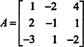The following row‐reduction operations, because they simply involve adding a multiple of one row to another, do not alter the value of the determinant:Now, when the determinant of this latter matrix is computed using the Laplace expansion by the first column, only one nonzero term remains: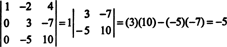Therefore, det A = −5.

Example 9: Evaluate the determinant of the matrixIn order to avoid generating many noninteger entries during the row‐reduction process, a factor of 2 is first divided out of the bottom row. Since multiplying a row by a scalar multiplies the determinant by that scalar,Now, because the elementary row operationsdo not change the determinant, Laplace expansion by the first column of this latter matrix completes the evaluation of the determinant of A: